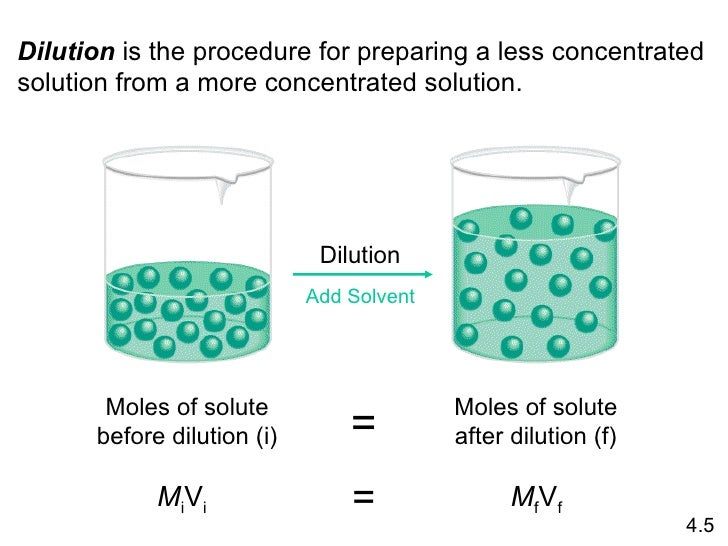# Question #c0425

Sep 10, 2017

(i) The number of moles does not change, but the molar concentration decreases.
(ii) The number of moles decreases, but the molar concentration does not change.

#### Explanation:

(i) Dilution

(a) Moles of solute

When you add more solvent, the volume of the solution increases but the number of particles stays the same.The number of moles of solute does not change.

(b) Molar concentration

The formula for molar concentration ($c$) is

$\textcolor{b l u e}{\overline{\underline{|}} \stackrel{\text{ ")(c = "moles"/"litres}}{|}}$

When you add more solvent, the volume increases but the number of particles stays the same.

Since "litres" is in the denominator, increasing the volume decreases the molar concentration.

(ii) Transferring some of the solution to another beaker

(a) Moles of solute

Let's say that you have 1 mol of solute in 1 L of solution, so $c = \text{1 mol/L}$.

When you transfer $\frac{1}{4}$ of the solution to another beaker, you have also
transferred $\frac{1}{4}$ of the particles.

Only $\frac{3}{4}$ of the particles remain in the first container.

∴ The number of moles decreases.

(b) Molar concentration

In the original beaker,

$c = \text{0.75 mol"/"0.75 L" = "1.0 mol/L}$

In the second beaker,

$c = \text{0.25 mol"/"0.25 L" = "1.0 mol/L}$

The molar concentration does not change.